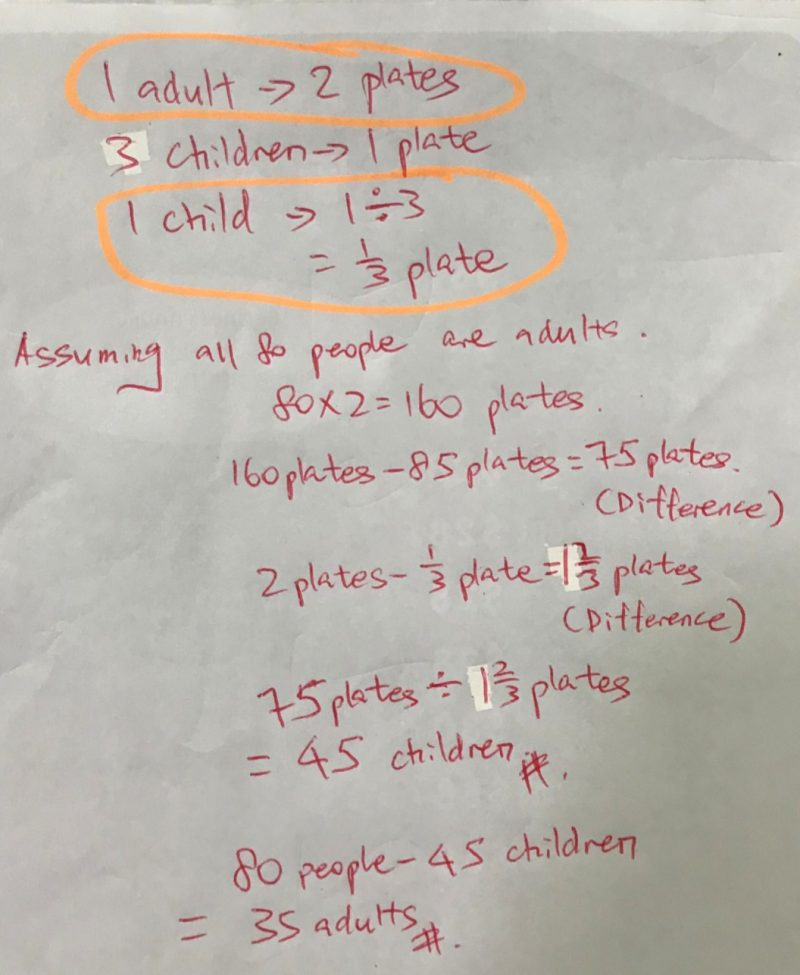# Question

How to solve this?

There are 85 plates of fried noodles for 80 people. Each adult eats 2 plates of fried noodles and every 3 children share 1 plate of fried noodles. How many adults and how many children are there?

Use algebra and simultaneous equations!

0 Replies 0 Likes

The method  taught in primary school to solve this type of question are supposition/Assumption and Guess n Check.

0 Replies 0 Likes

Once again the difficulty is to convert words into an equation 🙂
First, let’s say that:
C = number of children
We know that A + C = 80 (according to the wording). We have our first equation 🙂
Then we have to find the second one,
As every adult eats 2 plates and for 1 plate we need 3 children we have:

2A + (1/3)C = 85. We have our system 🙂

We know that : A= 80 – C

So we have then:
2*(80 – C)+ C/3 = 85
Which means:
C/3 – 2C = 85 – 160
Thus:
5/3C = 75

To conclude:
C = 45
We can now replace A in equation 1
A = 80 – 45 = 35

We have 35 adults and 45 children
Hope it will help 🙂

0 Replies 1 Like

number of (adults + children) ——- 80
number of adults x 2 + [(80 – number of adults)/3] x 1 ——- 85
6 x number of adults + (80 – number of adults) ——- 85 x 3 = 255
number of adults ——- (255 – 80)/5 = 35
80 – 35 = 45 (children)

Ans : 35 adults and 45 children.

Thanks

Don’t mention

0 Replies 0 Likes

Let adults is X and Child is Y

X:Y = 80

Total Plates: 85

Ratio:

2X :: Y/3 => 85

X::Y = 80 => 2X::2Y = 160

This can be solved as 2Y – Y/3 = 75

Y = 45

No. of children: 45